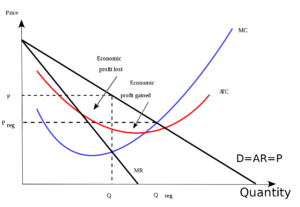# Profit Maximisation: Meaning, Producers Equilibrium, MC-MR Approach

The compilation of these The Theory of Firm Under Perfect Competition Notes makes students exam preparation simpler and organised.

## Profit Maximisation

For once step into the shoes of a producer and analyze your motive in economic terms. What keeps you burning your oil as a producer? Definitely, profit maximisation is your answer. A rational producer always looks from the monetary angle, trying to maximize his profits. Let us take a look at the economics behind profit maximisation.

### What is Profit?

Profit refers to the excess of receipts from the sale of goods over the expenditure incurred on producing them. The money received by a producer from the sale of his output is known as revenue. The money that goes into the process of production is known as cost. The difference between revenue and cost makes up the profit for a firm. So a rational producer would always prefer that his revenue column outshines the cost column in monetary terms.

### Producer’s Equilibrium and Profit Maximisation

Equilibrium represents a state of no change. Looking from a producer’s perspective, profit is their favorite word in the book of economics. Thus it is easy to realize that a producer would be in the state of equilibrium if he is earning a maximum profit, i.e has profit maximisation. Therefore producer’s equilibrium becomes our subject matter if we aim to understand profit maximisation.

A firm is said to be in equilibrium when it has no inclination to expand or to contract its output. This state either reflects profit maximisation or minimize losses. A producer can attain an equilibrium level under the following two situations:

Perfect competition: In the market conditions of perfect competition, a price is fixed by the industry which has to be accepted by all firms. Any quantity of the commodity can be sold at this price. Hence the price remains constant.

Imperfect competition: Under imperfect conditions, firms have the freedom to set price for their outputs. Note that sales can only be increased by reducing the price. In a nutshell, each firm follows an independent pricing policy which further means that the price is not constant.

### The Marginal Revenue-Marginal Cost Approach

Of course, profit depends on revenue and cost. As a result of this, the concept of producer equilibrium revolves around revenue and cost. According to the MR-MC approach, a producer is said to be in equilibrium when:

1. MR = MC
As long as the cost of producing another unit remains less than the revenue received from the sale of an additional unit, a producer won’t wander away from his path of earning profits. This is because, until MR > MC, it keeps on adding to profits. Production is stopped only when MR becomes equal to MC.

MR is the addition to TR from the sale of one more unit. MC is the addition to TC when an additional unit is produced. Thus when MR = MC, TR-TC becomes maximum for maximum profit. If MR exceeds MC, then the producer will continue producing as it will add to his profits.

On the contrary, if MR < MC then the benefit will be less than the cost. In essence, MR=MC is the only possible combination among the three to represent equilibrium.

2. MC is greater than MR after the MC = MR Output Level

When MC is greater than MR after equilibrium, the production of more units will lead a to decline in profits. MC can be equal to MR at more than one output level. In that case, if MC < MR after this level, it would not represent the equilibrium state as the producer would eye more profit.

Thus MR = MC is a necessary condition but not sufficient enough. It has to be supplemented by the condition that MR should be less than MC after this level, to ensure producer’s equilibrium.

Producer’s Equilibrium when Price remains ConstantUnder conditions of perfect competition, the price remains constant. We know that price is equal to AR. Further, the revenue from every additional unit is also equal to AR, when the price is constant. The AR curve coincides with the MR curve.

As explained we look for the level of output where MR becomes equal to MC (geometrically MR curve intersects with MC curve) and after this level, MC is greater than MR (geometrically the MC curve is above the MR curve after the equivalence point). When both these conditions are satisfied, the producer attains the equilibrium level of output.

Producer’s Equilibrium when Price is not ConstantUnder imperfect market conditions, the price tends to fall with an increase in output, as a producer can sell more units only at a lower price. The MR curve, in this case, slopes downwards. Producer aims to produce that level of output at which MC is equal to MR and after this level of output MC is greater than MR. This is depicted by the two curves intersecting each other and the MC curve rising above the MR curve past the intersection point.

Example:

Question:
What are the two necessary conditions that ensure producer’s equilibrium?